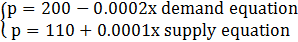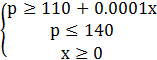### Sample Problem

The demand and supply equations for a new PDA are given bywhere p is the price (in dollars) and x represents the number of PDAs.

Find the consumer surplus and the producer surplus for these two equations.

Consumer surplus=

\$45,000,000

\$4,500,000

\$90,000,000

\$9,000,000

Producer surplus =

\$45,000,000

\$4,500,000

\$90,000,000

\$9,000,000

#### Solution

First, find the equilibrium point (the point at which supply and demand are equal) by solving the equation

200-0.0002x=110+0.0001x

90=0.0003x

X=300,000 PDAs, which corresponds to be an equilibrium of p=(110+0.0001(300,000))=\$140. So, the consumer surplus and producer surplus are the areas of the following regions =:

Consumer surplusProducer surplusAs shown in the graph, consumer and producer surpluses are defined as the areas of the shaded triangles. (The triangle shaded in green is the producer surplus and the triangle shaded in pink is the consumer surplus)

Consumer surplus=1/2bh=1/2(300,000)(200-140)=1/2(300,000)(60)=\$9,000,000

Producer surplus=1/2bh=1/2(300,000)(140-110)=1/2(300,000)(30)=\$4,500,000